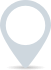# Basis point calculator

Use our handy basis point calculator to calculate the changes or differences in percentage terms. A basis point represents one-hundredth of a percentage point, or 0.01%.- %
bps

This calculator is intended for illustration purposes only and exact payment terms should be agreed with a lender before taking out a loan.

£
.00

What is x bps of y?

£-

## What is basis point and why is it used?

The calculator allows you to determine the numerical value of a given number of basis points, or to calculate the percentage change based on a given number of basis points.

The primary purpose of a basis point calculator is to simplify financial calculations and comparisons, particularly in the realm of finance, investments, and interest rates. It is commonly used in the following scenarios:

1. Interest Rates: When comparing different interest rates or analyzing changes in interest rates, basis points are used to express the difference. For example, if the interest rate increases by 25 basis points, it means the rate has increased by 0.25%.

2. Bond Yields: Basis points are frequently used to measure changes in bond yields. A basis point calculator helps determine the change in yield when comparing different bonds or analyzing market fluctuations.

3. Financial Spreads: In finance, spreads often refer to the difference between two interest rates or bond yields. By using basis points, the spread can be expressed and compared more precisely.

4. Investment Returns: When evaluating investment performance, basis points can be used to express changes in returns. For example, if an investment’s return increased by 200 basis points, it means the return increased by 2%.

By using a basis point calculator, financial professionals and individuals can easily quantify and compare small changes in percentages, making it a valuable tool for analyzing and understanding financial data.

### Clever finance tips and the latest news

#### delivered to your inbox, every week